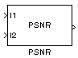PSNR

Compute peak signal-to-noise ratio (PSNR) between images

• Library:
• Computer Vision Toolbox / Statistics

•Description

The PSNR block computes the peak signal-to-noise ratio, in decibels, between two images. This ratio is used as a quality measurement between the original and a compressed image. The higher the PSNR, the better the quality of the compressed, or reconstructed image.

The mean-square error (MSE) and the peak signal-to-noise ratio (PSNR) are used to compare image compression quality. The MSE represents the cumulative squared error between the compressed and the original image, whereas PSNR represents a measure of the peak error. The lower the value of MSE, the lower the error.

To compute the PSNR, the block first calculates the mean-squared error using the following equation:

$MSE=\frac{\sum _{M,N}{\left[{I}_{1}\left(m,n\right)-{I}_{2}\left(m,n\right)\right]}^{2}}{M*N}$

In the previous equation, M and N are the number of rows and columns in the input images. Then the block computes the PSNR using the following equation:

$PSNR=10{\mathrm{log}}_{10}\left(\frac{{R}^{2}}{MSE}\right)$

In the previous equation, R is the maximum fluctuation in the input image data type. For example, if the input image has a double-precision floating-point data type, then R is 1. If it has an 8-bit unsigned integer data type, R is 255, etc.

Computing PSNR for Color Images

Different approaches exist for computing the PSNR of a color image. Because the human eye is most sensitive to luma information, you can compute the PSNR for color images by converting the image to a color space that separates the intensity (luma) channel, such as YCbCr. The Y (luma), in YCbCr represents a weighted average of R, G, and B. G is given the most weight, again because the human eye perceives it most easily. Compute the PSNR only on the luma channel.

Ports

Input

expand all

Input image, specified as scalar, vector, or matrix.

Data Types: single | double | int8 | int16 | int32 | int64 | uint8 | uint16 | uint32 | uint64 | Boolean | fixed point

Input image, specified as scalar, vector, or matrix.

Data Types: single | double | int8 | int16 | int32 | int64 | uint8 | uint16 | uint32 | uint64 | Boolean | fixed point

Output

expand all

Peak signal-to-noise ratio between images, returned as a scalar.

Dependencies

If the input is a fixed-point or integer data type, the block output is double-precision floating point. Otherwise, the block input and output are the same data type.

Data Types: double

Block Characteristics

 Data Types double | fixed point | integer | single Multidimensional Signals no Variable-Size Signals yes